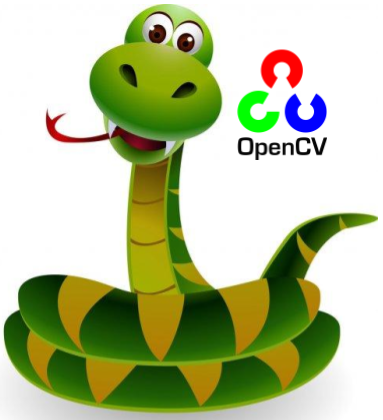# Python卡通化照片【浅阅篇】

二维码 541. 加载图片
1. 创建边缘掩膜
1. 减少调色板
1. 将边缘掩膜与彩色图像结合

## 加载图片

``````def read_file(filename):
cv2_imshow(img)
return img``````

``````uploaded = files.upload()

## 创建边缘掩膜

``````def edge_mask(img, line_size, blur_value):
gray = cv2.cvtColor(img, cv2.COLOR_BGR2GRAY)
gray_blur = cv2.medianBlur(gray, blur_value)
return edges``````

``````line_size = 7
blur_value = 7
cv2_imshow(edges)``````

## 减少调色板

### 颜色量化

``````def color_quantization(img, k):
# Transform the image
data = np.float32(img).reshape((-1, 3))

# Determine criteria
criteria = (cv2.TERM_CRITERIA_EPS + cv2.TERM_CRITERIA_MAX_ITER, 20, 0.001)

# Implementing K-Means
ret, label, center = cv2.kmeans(data, k, None, criteria, 10, cv2.KMEANS_RANDOM_CENTERS)
center = np.uint8(center)
result = center[label.flatten()]
result = result.reshape(img.shape)
return result``````

``````total_color = 9
img = color_quantization(img, total_color)``````

### 双边过滤器

``blurred = cv2.bilateralFilter(img, d=7, sigmaColor=200,sigmaSpace=200)``

• d —每个像素邻域的直径。
• sigmaColor —参数的值越大，表示面积越大的半均等颜色。
• sigmaSpace –该参数的值越大，表示越远的像素就会相互影响，只要它们的颜色足够接近即可。

## 结合边缘掩膜和彩色图像

``cartoon = cv2.bitwise_and(blurred, blurred, mask=edges)``